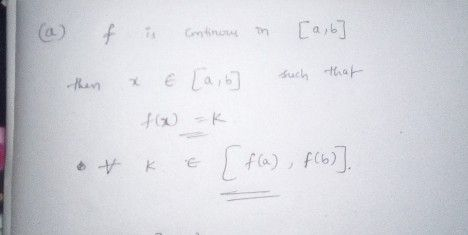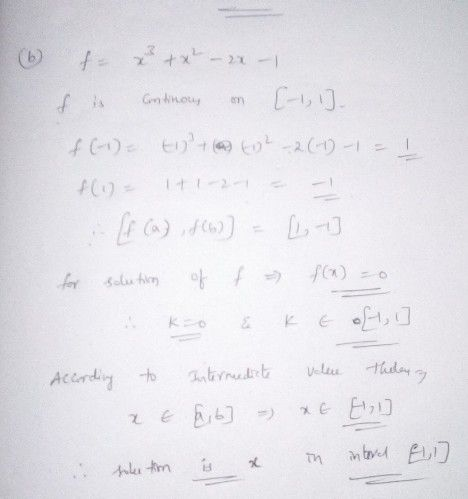Symbol
Problem$\left(4ma\pi ks\right)$ $2$ The Intermediate-Value Theorem states that if $4$ function $f$ is continuous on closed interval $\left(a,b\right)$ and k is any number between $f\left(a\right)$ and $f\left(b\right)$ inclusive, then there is at least one number $x$ in the interval $a.b$ such that $f\left(x\right)=k$ $\left(a\right)$ Give a geometrical interpretation of the Intermediate-Value Theorem. (b) Use the Intermediate-Value Theorem to show that the equation $x^{3}+x^{2}-2x=1$ has at least one solution in the $intarma1$ $-1$ $1\right)$ $\left(2+3=5ma\pi ks\right)$
Calculus
SolutionQanda teacher - Nivas